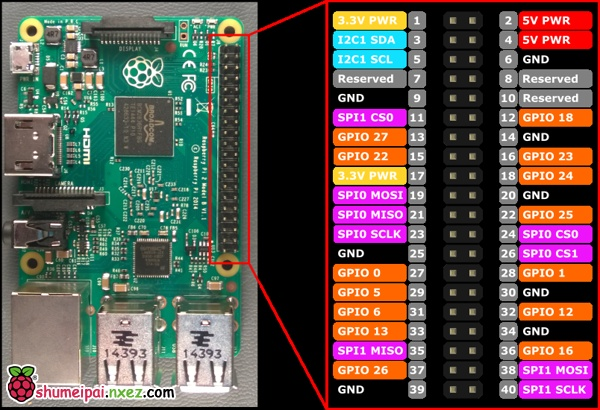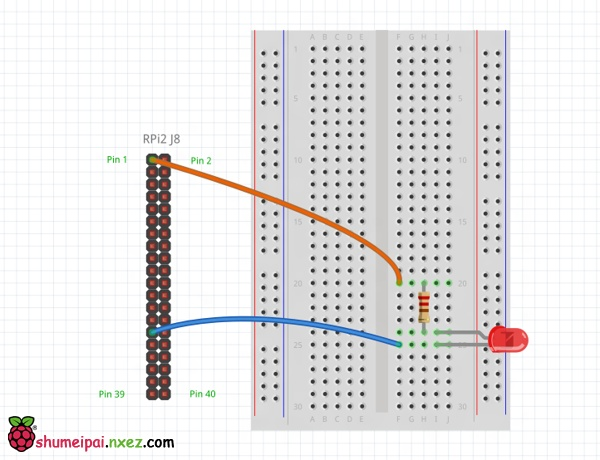# 树莓派 Windows10 IoT Core 开发教程 LED

#### 将LED连接到 Windows 10 设备#### 部署应用程序#### 定时器代码

 1 2 3 4 5 6 7 8 9 10 11 12 13 14 15 16 `public` `MainPage()` `{` `    ``// ...` `    ``this``.timer = ``new` `DispatcherTimer();` `    ``this``.timer.Interval = TimeSpan.FromMilliseconds(500);` `    ``this``.timer.Tick += Timer_Tick;` `    ``this``.timer.Start();` `    ``// ...` `}` `private` `void` `Timer_Tick(``object` `sender, ``object` `e)` `{` `    ``FlipLED();` `}`

#### 初始化GPIO引脚

 1 2 3 4 5 6 7 8 9 10 11 12 13 14 15 16 17 18 19 20 21 22 23 24 25 26 27 28 `using` `Windows.Devices.Gpio;` `private` `void` `InitGPIO()` `{` `    ``var` `gpio = GpioController.GetDefault();` `    ``// Show an error if there is no GPIO controller` `    ``if` `(gpio == ``null``)` `    ``{` `        ``pin = ``null``;` `        ``GpioStatus.Text = ``"There is no GPIO controller on this device."``;` `        ``return``;` `    ``}` `    ``pin = gpio.OpenPin(LED_PIN);` `    ``// Show an error if the pin wasn't initialized properly` `    ``if` `(pin == ``null``)` `    ``{` `        ``GpioStatus.Text = ``"There were problems initializing the GPIO pin."``;` `        ``return``;` `    ``}` `    ``pin.Write(GpioPinValue.High);` `    ``pin.SetDriveMode(GpioPinDriveMode.Output);` `    ``GpioStatus.Text = ``"GPIO pin initialized correctly."``;` `}`

～首先，使用GpioController.GetDefault()获取GPIO控制权限
～如果设备不具有可用的GPIO资源，则返回null
～接下来通过调用GpioController.OpenPin()函数来打开GPIO引脚
～当我们获取了GPIO的控制权限并打开了GPIO引脚后，使用GpioPin.Write()函数来将LED关闭（参数设置High）
～这里还使用了GpioPin.SetDriveMode()函数将GPIO引脚的工作模式设置为输出模式。

#### 改变GPIO引脚的状态

this.pin.Write(GpioPinValue.Low);

this.pin.Write(GpioPinValue.High);